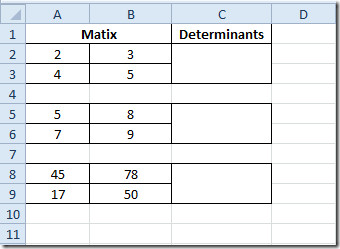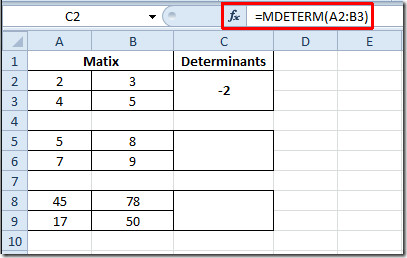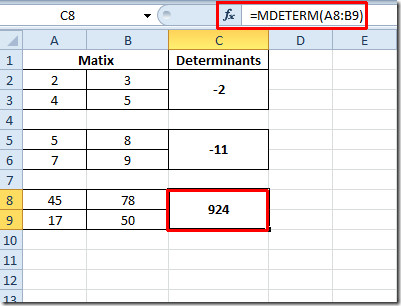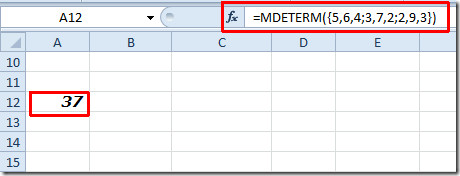# Excel 2010 Evaluating Matrix Determinant (MDETERM Function)

Apart from supporting statistical and financial functions, Excel also contains some advance mathematical  functions. Matrix operations have been widely used for various purposes. Excel MDETERM function allow users to find out the determinant of given matrix. It has made evaluating determinant dead-easy for users working with matrices.

Launch Excel 2010 spreadsheet on which you want to apply Matrix determinant. For instance, we have included a spreadsheet containing fields Matrix and Determinants.You might have been acquaint with the orthodox way of finding out matrix determinant.

|A| = (a*b)-(c*d)

Where a,b,c, and d are the elements stored in matrix. With Excel built-in function we don’t need to write in lengthy formula to calculate the determinant of matrix. The MDETERM function will allow us to evaluate determinant instantly.

The syntax of this function is;

=MDETERM(array)

It requires single argument in the form of array. In our case array is matrix containing integers.

We will be writing this formula as

=MDETERM(A2:B3)

The argument in the function is A2:B3, which is the location of the cells containing elements of matrix. We will be writing this formula in first row of Determinants field. It yields –2 by evaluating the whole formula, as shown in the screenshot below.Now we will be applying this formula on the Determinants column, just copy the cell containing formula, and paste it into other cells. The cell location will change automatically on pasting the formula in other cells.If you want to evaluate determinant by specifying values directly, then you need to write it as;

==Mdeterm({5,6,4;3,7,2;2,9,3})You can also check out previously reviewed Excel Functions; DOLLAR, SUMPRODUCT, SUMIF, COUNTIF, VLOOKUP, HLOOKUP ,PMT, and LEN.

1.hasenmaus says: